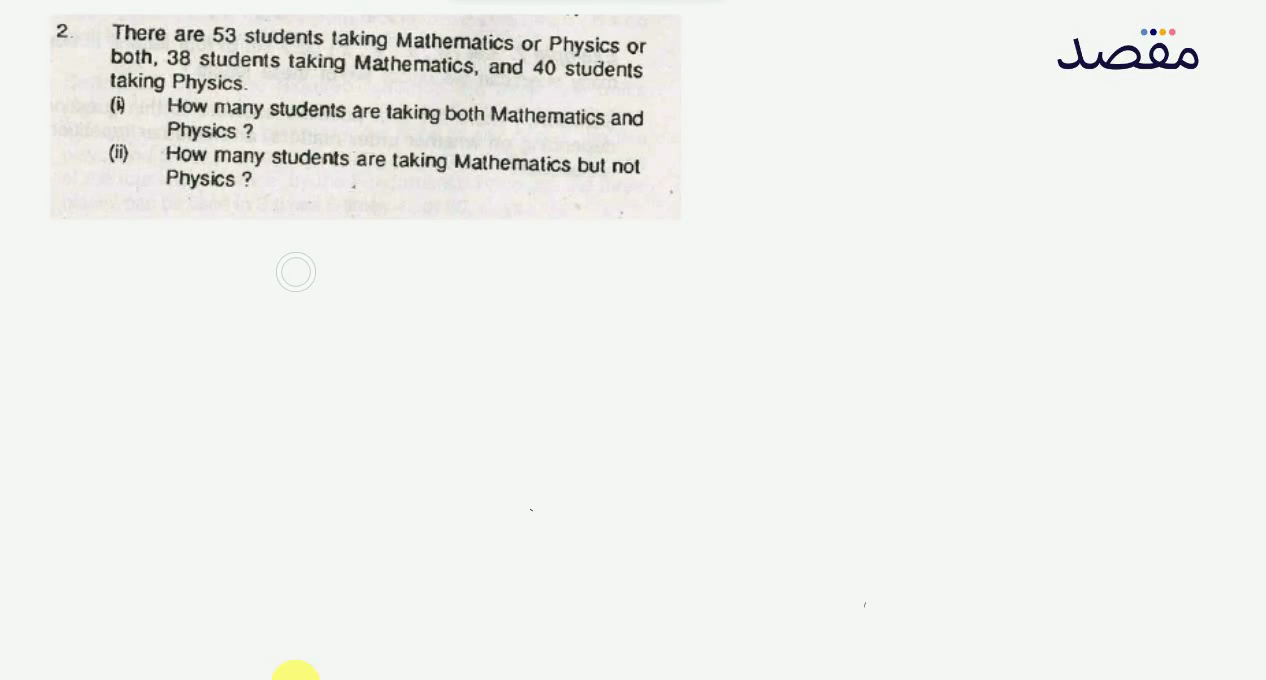# Classes

Change the way you learn with Maqsad's classes. Local examples, engaging animations, and instant video solutions keep you on your toes and make learning fun like never before!

Class 9Class 10First YearSecond Year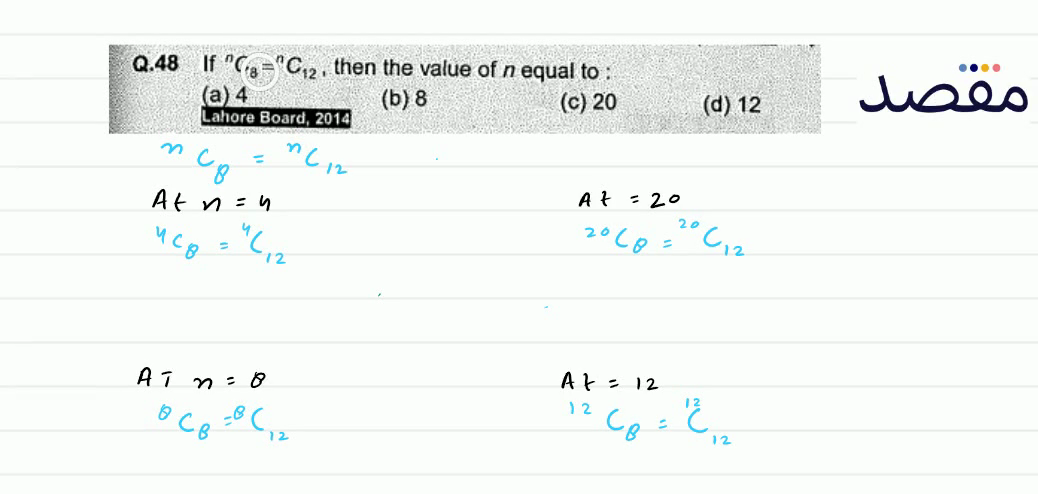### Q.48 If { }^{n} C_{3}={ }^{n} C_{12} then the value of n equal to :(a) 4(b) 8(c) 20(d) 12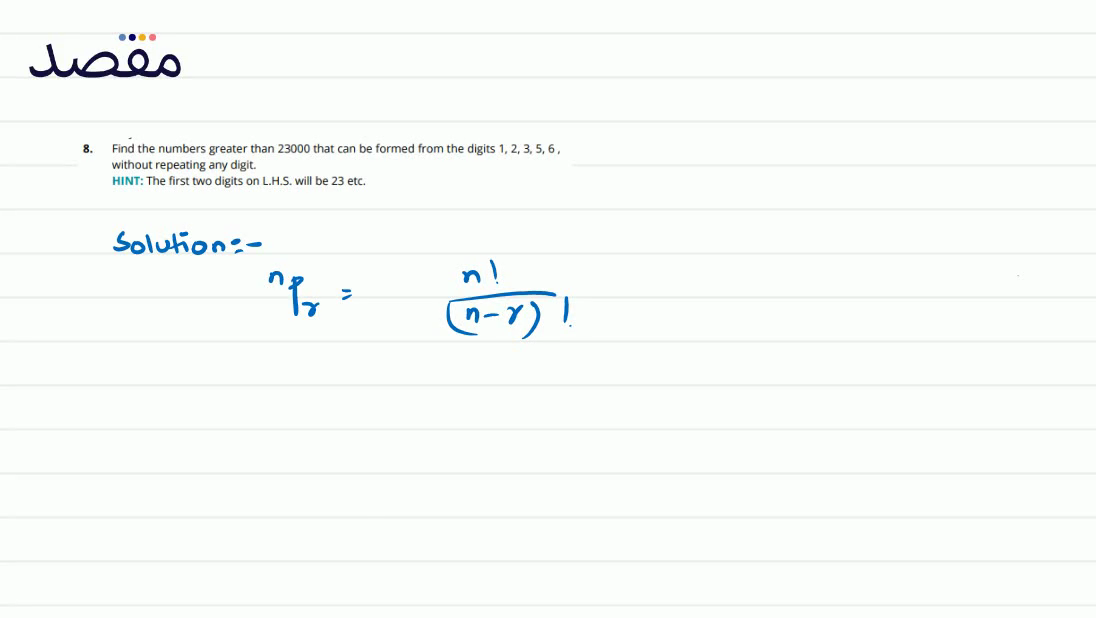### 8. Find the numbers greater than 23000 that can be formed from the digits 12356 without repeating any digit.HINT: The first two digits on L.H.S. will be 23 etc.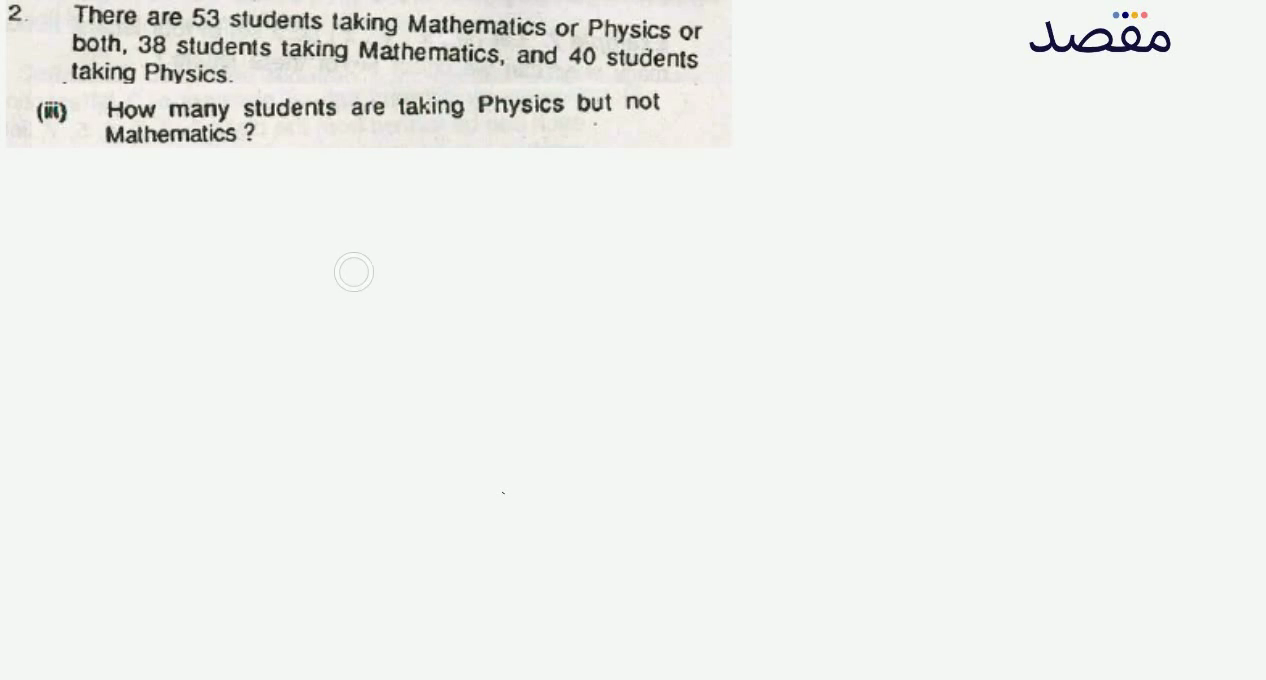### 2. Write each of the following in the factorial form:iii) 20.19 .18 .17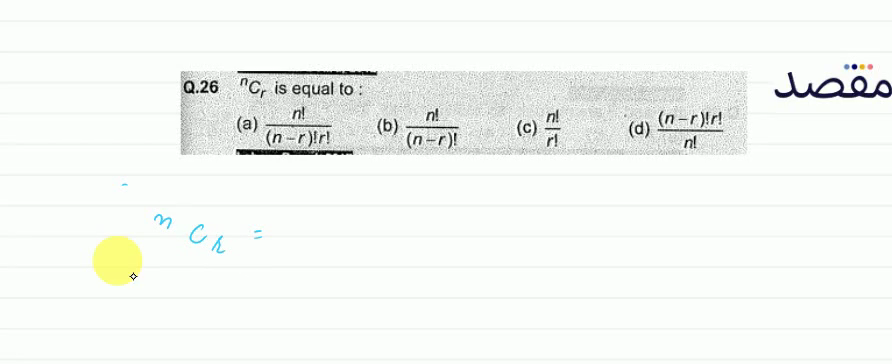### Q.26 { }^{n} C_{r} is equal to :(a) \frac{n !}{(n-r) ! r !} (b) \frac{n !}{(n-r) !} (c) \frac{n !}{r !} (d) \frac{(n-r) ! r !}{n !}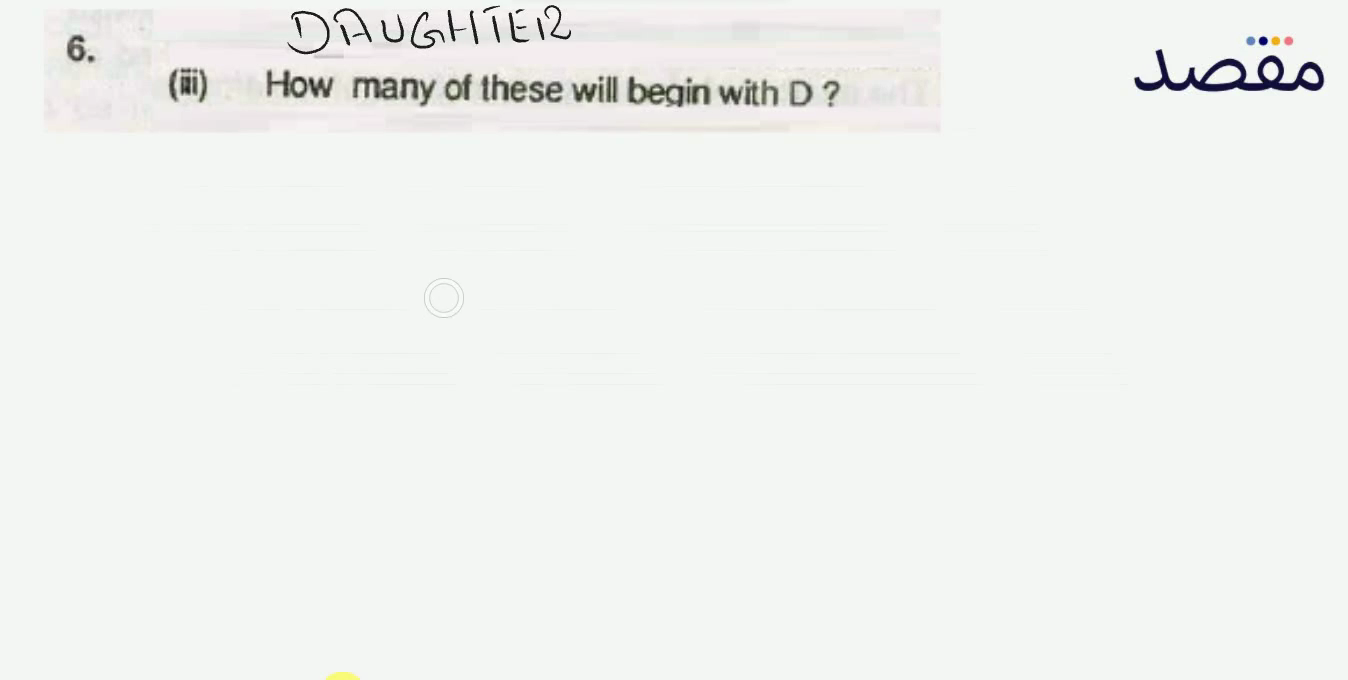### 6. How many words can be formed from the letters of the following words using all letters when no letter is to be repeated:iii) FASTING?